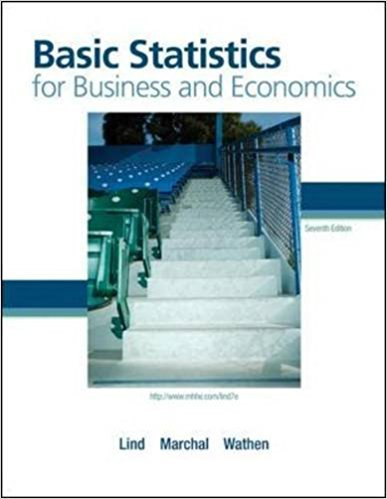×
Get Full Access to Basic Statistics For Business And Economics - 7 Edition - Chapter 2 - Problem 27e
Get Full Access to Basic Statistics For Business And Economics - 7 Edition - Chapter 2 - Problem 27e

×

# A data set consists of 83 observations. How many classesISBN: 9780077384470 64

## Solution for problem 27E Chapter 2

Basic Statistics for Business and Economics | 7th Edition

• Textbook Solutions
• 2901 Step-by-step solutions solved by professors and subject experts
• Get 24/7 help from StudySoup virtual teaching assistantsBasic Statistics for Business and Economics | 7th Edition

4 5 1 259 Reviews
19
0
Problem 27E

Problem 27E

A data set consists of 83 observations. How many classes would you recommend for a frequency distribution?

Step-by-Step Solution:
Step 1 of 3

Solution 27E

Step1 of 2:

From the given problem we have total number of observations = 83.

We need to find the classes for a frequency distribution.

Step2 of 2:

We have totally 83 observations, by using 2 to the power ‘k’ rule.

The 25 = 32, which is less than 83, so 5 classes are not enough.

Take the 26 = 64, which is less than 83, so 6 classes are not enough.

Take the 27 = 128, which is greater than 83.

Hence, I will recommend 7 classes.

Step 2 of 3

Step 3 of 3

##### ISBN: 9780077384470

The answer to “A data set consists of 83 observations. How many classes would you recommend for a frequency distribution?” is broken down into a number of easy to follow steps, and 17 words. Basic Statistics for Business and Economics was written by and is associated to the ISBN: 9780077384470. This full solution covers the following key subjects: classes, consists, data, distribution, frequency. This expansive textbook survival guide covers 6 chapters, and 325 solutions. The full step-by-step solution to problem: 27E from chapter: 2 was answered by , our top Business solution expert on 08/23/17, 08:36AM. Since the solution to 27E from 2 chapter was answered, more than 437 students have viewed the full step-by-step answer. This textbook survival guide was created for the textbook: Basic Statistics for Business and Economics , edition: 7.

Unlock Textbook Solution